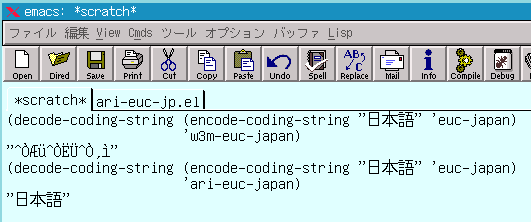# Re: pccl

```CCL \$B\$OJ,\$+\$C\$F\$\$\$J\$\$\$N\$G\$9\$,!"(B`write-multibyte-character' \$B\$r(B
\$B;H\$&\$H(B XEmacs \$B\$G\$b2=\$1\$J\$\$\$h\$&\$G\$9!#(B

\$BE,Ev\$K(B w3m-euc-japan \$B\$r4p\$K!"(Bari-euc-japan \$B\$C\$F\$N\$r=q\$\$\$F\$_\$^\$7\$?\$,!"(B
jisx0208 \$B\$K4X\$7\$F\$O2=\$1\$J\$\$\$3\$H\$r3NG'\$7\$^\$7\$?!#(B
``````(require 'pccl)
(require 'w3m)

(define-ccl-program ari-euc-japan-decoder
`(2
(loop
;; Process normal EUC characters.
(if (r0 < #x80)
(write-repeat r0))
(if (r0 > #xa0)
(r1 &= #x7f)
(r1 |= ((r0 & #x7f) << 7))
(r0 = ,(charset-id 'japanese-jisx0208))
(write-multibyte-character r0 r1)
(repeat)))
(if (r0 == #x8e)
(r0 = ,(charset-id 'katakana-jisx0201))
(write-multibyte-character r0 r1)
(repeat)))
(if (r0 == #x8f)
(r1 &= #x7f)
(r1 |= ((r0 & #x7f) << 7))
(r0 = ,(charset-id 'japanese-jisx0212))
(write-multibyte-character r0 r1)
(repeat)))
;; Process internal characters used in w3m.
(if (r0 == ?\x80)			; Old ANSP (w3m-0.1.11pre+kokb23)
(write-repeat 32))
(if (r0 == ?\x90)			; ANSP (use for empty anchor)
(write-repeat 32))
(if (r0 == ?\x91)			; IMSP (blank around image)
(write-repeat 32))
(if (r0 == ?\xa0)			; NBSP (non breakble space)
(write-repeat 32))
(write-repeat r0))))

(make-ccl-coding-system
'ari-euc-japan ?E
"ISO 2022 based EUC encoding for Japanese with w3m internal characters."
'ari-euc-japan-decoder 'w3m-euc-japan-encoder)
```
```--
\$BM-Bt(B \$BL@9((B
```

• Namazu Search: [Help]